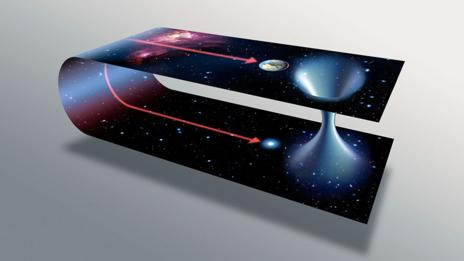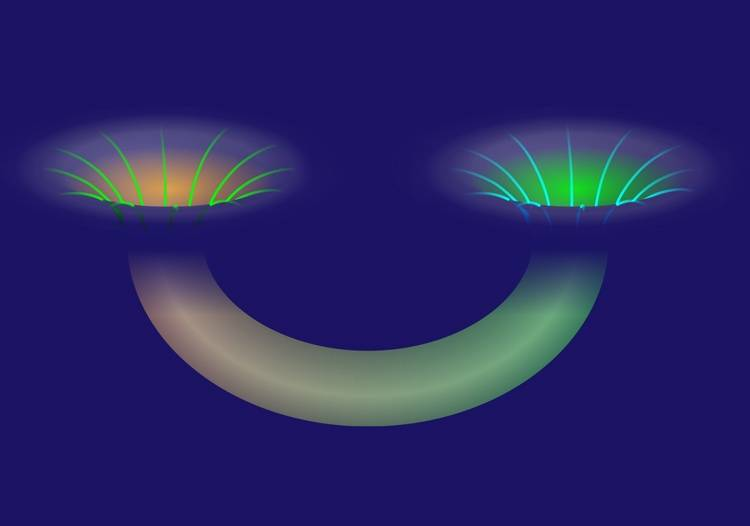# Prerequisites for a wormhole

Gold Member
Still reading Kip Thorne's book on Interstellar. It, like so many other books that describe wormholes, shows a schematic of a wormhole like this:It's easy to intuit that the path through the wormhole is much shorter than the path through "normal" space. But that's because they're drawn it such that normal space must curve back on itself quite dramatically.

Is this curvature of normal space a real property required for the wormhole to be a shorter distance?

Contrast with this simple diagram:This depicts flat space, with no huge curvature.
If one interprets it literally, the path through the wormhole is significantly longer.

So, back to the FIRST image, my question is: is the curvature depicted in the topmost diagram to be interpreted as a real curvature of space, and does it have to be in existence before the wormhole is created (i.e. independent of the WH)? This leading to the obvious question: it is likely such a curvature even exists somewhere, let alone somewhere useful to us?

Ultimately, it seems to me that, while wormholes might be hypothetically possible, we could in fact roam the universe for a cosmological age before ever finding a place with enough curvature that a wormhole could take advantage of (except perhaps within the gravity well of a black hole, where curvature is so great that we might be able to take a shortcut across an arc, from one side to another. But really, why?)

Last edited:

PeterDonis
Mentor
is the curvature depicted in the topmost diagram to be interpreted as a real curvature of space, and does it have to be in existence before the wormhole is created

The straightforward answer is no: the "distance" in the background "hyperspace" of the topmost diagram does not correspond to anything physical. The diagram is drawn that way for convenience in visualization, nothing more. For example, we could just as easily draw a diagram with normal space being flat and the wormhole "curving" in the background hyperspace between two widely separated points in normal space, and then just saying that, when going through the wormhole, a very long distance in the background hyperspace corresponds to a much shorter distance through the wormhole (whereas the correspondence is assumed to be 1 to 1 in normal space). Nothing in the math of GR prevents that.

That said, I'm actually not sure if anyone has explored what, if any, limitations there are in the actual physical model, i.e., what, if any, limitations there are on the curvature in normal space between two points that are connected by a wormhole. Some of the other experts here might know of relevant literature on this, but I don't.

martinbn
The curvature that you see on the picture is just an artifact of the picture. The space is actually flat, where the "sheet" looks bent. The embedding of this surface in three space is not isometric i.e. the metric is not the one induced from the Euclidean metric of the three space. When you see it curved it is because your intuition uses the usual induced metric.

Orodruin
Staff Emeritus
Homework Helper
Gold Member
2021 Award
The embedding of this surface in three space is not isometric i.e. the metric is not the one induced from the Euclidean metric of the three space.

It might very well be the induced metric. However, the intrinsic curvature can still be zero, just like the curvature of a cylinder embedded in R3 with the induced metric:
$$ds^2 = dz^2 + R^2 d\phi^2$$
which is most definitely flat.

Gold Member
For example, we could just as easily draw a diagram with normal space being flat and the wormhole "curving" in the background hyperspace between two widely separated points in normal space.
The space is actually flat, where the "sheet" looks bent.

Shoot. My second image in the opening post did not show up. I have edited the opening post to point to a different image which hopefully this time will render.

I take it, if you guys had seen it, your responses might have been a simple "Yes to option B". ;)

PeterDonis
Mentor
I take it, if you guys had seen it, your responses might have been a simple "Yes to option B". ;)

Yes, as long as option B includes the fact that the actual distance you have to travel through the wormhole is shorter (i.e., the "distances" shown in the diagram, the "distances in hyperspace", don't necessarily match up with actual physical distances).

martinbn
It might very well be the induced metric. However, the intrinsic curvature can still be zero, just like the curvature of a cylinder embedded in R3 with the induced metric:
$$ds^2 = dz^2 + R^2 d\phi^2$$
which is most definitely flat.

You are right.

Gold Member
Yes, as long as option B includes the fact that the actual distance you have to travel through the wormhole is shorter (i.e., the "distances" shown in the diagram, the "distances in hyperspace", don't necessarily match up with actual physical distances).
Right. Diagram 2 in my opening post is equally as inaccurate as diagram 1. Both represent some part of space as being longer than it would be if it were traversed.

PeterDonis
Mentor
Both represent some part of space as being longer than it would be if it were traversed.

Actually, diagram 1 represents lengths correctly (or at least it can be stipulated to do so); what it misrepresents is spatial curvature. Diagram 2 misrepresents, at a minimum, the spatial length through the wormhole; it may also misrepresent spatial curvature through the wormhole (if we assume that spacelike lines through the wormhole are actually straight, as they appear in diagram 1).